Derivative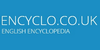A derivative is a security whose value is 'derived' from the performance or movement of another financial security, index or other investment. For example, derivatives may be futures

DerivativeThe derivative of a function of a real variable measures the sensitivity to change of a quantity (a function or dependent variable) which is determined by another quantity (the independent variable). It is a fundamental tool of calculus. For example, the derivative of the position of a moving object with respect to time is the object`s velocity: ....
Found on http://en.wikipedia.org/wiki/Derivative

Derivative• (n.) A chord, not fundamental, but obtained from another by inversion; or, vice versa, a ground tone or root implied in its harmonics in an actual chord. • (n.) That which is derived; anything obtained or deduced from another. • (n.) A substance so related to another substance by modification or partial substitution as to be regard...
Found on http://thinkexist.com/dictionary/meaning/derivative/

derivativeadjective resulting from or employing derivation; `a derivative process`; `a highly derivative prose style`
Found on https://www.encyclo.co.uk/local/20974

derivative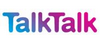(maths) In mathematics, the limit of the gradient of a chord linking two points on a curve as the distance between the points tends to zero; for a function of a single variable, y = f(x), it is denoted by f ...
Found on http://www.talktalk.co.uk/reference/encyclopaedia/hutchinson/m0026030.html

Derivative[chemistry] In chemistry, a derivative is a compound that is derived from a similar compound by some chemical or physical process. In the past it was also used to mean a compound that can be imagined to arise from another compound, if one atom is replaced with another atom or group of atoms, but modern chemical language now uses the term st...
Found on http://en.wikipedia.org/wiki/Derivative_(chemistry)

Derivative[finance] In finance, a derivative is a special type of contract that derives its value from the performance of an underlying entity. This underlying entity can be an asset, index, or interest rate, and is often called the `underlying`. Derivatives can be used for a number of purposes - including insuring against price movements (hedging), ...
Found on http://en.wikipedia.org/wiki/Derivative_(finance)

DerivativeDe·riv'a·tive adjective [ Latin derivativus : confer French dérivatif .] Obtained by derivation; derived; not radical, original, or fundamental; originating, deduced, or formed from something else; secondary; as, a derivative conveyance; a derivative word. Derivative ...
Found on http://www.encyclo.co.uk/webster/D/43

derivativeA chemical substance derived from another substance either directly or by modification or partial substitution. ... (18 Nov 1997) ...
Found on http://www.encyclo.co.uk/local/20973

derivative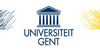a chemical substance derived from another substance either directly or by modification or partial substitution.
Found on http://users.ugent.be/~rvdstich/eugloss/DIC/dictio24.html

Derivative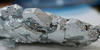A collective term for securities whose prices are based on the prices of another underlying investment. Derivatives are essentially a bet on which way the price of the underlying instrument is going and can be used to reduce the risk of (hedge) and investment in the underlying instrument.
Found on http://www.insidemetals.com/index.php?view=mining_glossary

derivative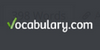a compound obtained from another compound
Found on https://www.vocabulary.com/lists/388588

derivative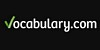a compound obtained from another compound
Found on https://www.vocabulary.com/lists/173764

Derivative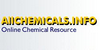A compound that can be imagined to arise from a partent compound by replacement of one atom with another atom or group of atoms. Used extensively in orgainic chemistry to assist in identifying compounds.
Found on http://www.encyclo.co.uk/local/20728

Derivative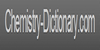A compound that can be imagined to arise from a partent compound by replacement of one atom with another atom or group of atoms. Used extensively in orgainic chemistry to assist in identifying compounds.
Found on http://www.chemistry-dictionary.com/definition/derivative.php

Derivative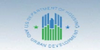a contract between two or more parties where the security is dependent on the price of another investment.
Found on https://www.encyclo.co.uk/local/21674

DerivativeA financial contract that derives its value from an underlying security, liability or index. Derivatives come in many varieties, including forwards, futures, options, warrants and swaps. Also known as Synthetic.
Found on http://www.encyclo.co.uk/local/20174

Derivative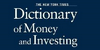A financial contract whose value is based on, or 'derived' from, a traditional security (such as a stock or bond), an asset (such as a commodity), or a market index.
Found on http://www.duke.edu/~charvey/Classes/wpg/bfglosd.htm

Derivative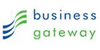A financial contract with a value linked to the expected future price movements of the asset it is linked to - such as a share or a currency.
Found on http://www.encyclo.co.uk/local/20546

Derivative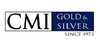A financial instrument derived from a cash market commodity, futures contract, or other financial instrument. Derivatives can be traded on regulated exchanges or over-the-counter. Futures contracts, for example, are derivatives of physicals commodities, and options on futures are derivatives of futures contracts.
Found on http://www.cmi-gold-silver.com/precious-metals-glossary-of-terms/

Derivative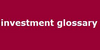A financial instrument whose price and performance is linked to that of an underlying security.
Found on http://www.encyclo.co.uk/local/20416

Derivative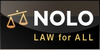A financial instrument whose value is based on the value of an underlying security, such as a commodity, currency, or bond. The most common derivatives are futures, options, and swaps. They are used to manage risk and fluctuations in the value of the underlying security but are often risky and complicated investments.
Found on http://www.nolo.com/dictionary/derivative-term.html

derivativeA financial structure such as a future or and option which derives from another financial asset. Thus for example equity derivatives derive from underlying equities.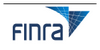A generic term often applied to a wide variety of financial instruments that derive their cash flows, and therefore their value, by reference to an underlying asset, reference rate, or index.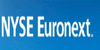A security whose value is dependent on, or derived from, the value of some underlying asset.